# Geometry of Hydrogen Atom Solution

The hydrogen atom solution to the Schrodinger equation produces three quantum numbers which can be seen to arise naturally from geometrical constraints on the wavefunction.Separated into equations in terms of the spherical coordinates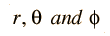the wavefunction takes the formwhich gives three equations. The equation for each of the three variables gives rise to a quantum number and the quantized energy states of the atom can be specified in terms of these quantum numbers.
Index

Hydrogen concepts

 HyperPhysics***** Quantum Physics R Nave
Go Back

# Quantum Numbers, Hydrogen Atom

In the solution to the Schrodinger equation for the hydrogen atom, three quantum numbers arise from the space geometry of the solution and a fourth arises from electron spin. No two electrons can have an identical set of quantum numbers according to the Pauli exclusion principle , so the quantum numbers set limits on the number of electrons which can occupy a given state and therefore give insight into the building up of the periodic table of the elements.R(r)   Principal quantum number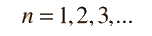P(θ) Orbital quantum number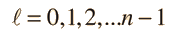F(φ) Magnetic quantum number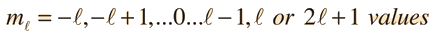Spin quantum number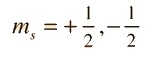Quantum numbers and atomic energy levels
Index

Hydrogen concepts

 HyperPhysics***** Quantum Physics R Nave
Go Back